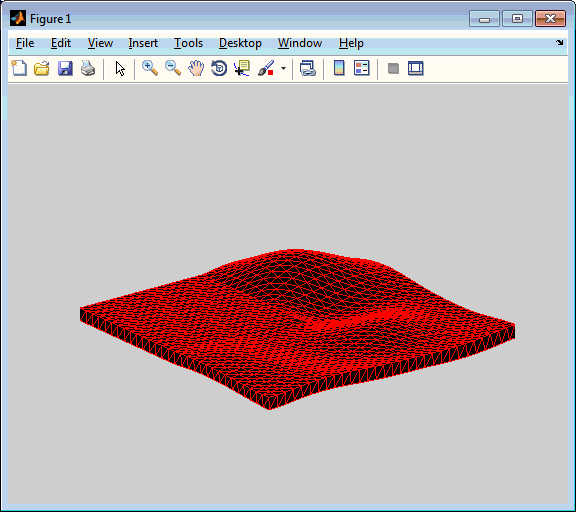# Surface To Solid

Jiro's pick this week is surf2solid by Sven.

Sven is no stranger to Pick of the Week. His inpolyhedron was Picked by Sean recently, and he's also active in other areas of MATLAB Central. In fact, this entry was inspired by a post written by my colleague Paul on "Printing the 3D L-Shaped Membrane".

3D Printing allows you to easily create 3D objects from a digital model, such as models created in MATLAB. People are already using MATLAB for 3D printing, and we've written about it a few times, including the post above by Paul, as well as "Printing Math" and "Anamorphic 3D Printing".

In order to create a 3D object, we can't print a surface which has no thickness. Paul's post above talks about the process of adding that thickness. Sven took some of those techniques and created surf2solid. It's very easy to use with very good instructions (help). You simply provide the data that you would normally pass into surf or patch, and optionally specify the thickness (for a uniform thickness), the elevation (for a block with a flat bottom), and the triangulation method.

Since I don't have one of those fancy 3D printing machines, I'll show an animation of a vibrating (thick) membrane. This is a modification of a shipping demo called vibes.

% Eigenvalues
lambda = [9.6397238445, 15.19725192, 2*pi^2, ...
29.5214811, 31.9126360, 41.4745099, 44.948488, ...
5*pi^2, 5*pi^2, 56.709610, 65.376535, 71.057755];

% Eigenfunctions
for k = 1:12
L{k} = membrane(k);
end

% Get coefficients from eigenfunctions
for k = 1:12
c(k) = L{k}(25,23)/3;
end

% Construct solids for animation
S = struct('faces',cell(1,500),'vertices',cell(1,500));
x = (-15:15)/15;
t = 0;
for id = 1:length(S)
% Coefficients
t = t + 0.02; % increment time
s = c.*sin(sqrt(lambda)*t);

% Amplitude
A = zeros(size(L{1}));
for k = 1:12
A = A + s(k)*L{k};
end

% Convert SURF data to SOLID data
S(id) = surf2solid(x,x,A,'thickness',-0.1);
end

% Create patch and modify view
pHandle = patch(S(1),'EdgeColor','r');
view(3)
axis([-1 1 -1 1 -.5 1])
axis off

% Animate
for id = 1:length(S)
set(pHandle,'Faces',S(id).faces,'Vertices',S(id).vertices);
drawnow
end